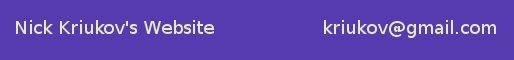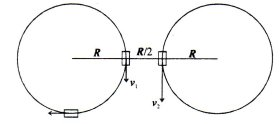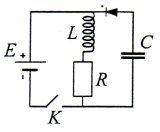Nick Kriukov's Website| Home | Physics | Computers | Languages | About Me | Misc |

 1. (8 points) Biker races are performed on a narrow circular track. Every biker tries to achieve big speed as faster as possible right after the start. Which part of the circle does he cover before he achieves it?2. (12 points) Cars A and B move on the tracks which are horizontal circles of the same radius lying in the same plane and whose closest points are apart by half the radius. The velocity of car A is v1 = 20 km/h, of car B is v2 = 40 km/h. Find the velocity of car B relative to car A at the moment when they are at the closest points and when car A made a quarter of the revolution after that. 3. (8 points) A little bulb with remaining freon was connected to a large reservoir in which the initial pressure p was twice as big as in the bulb and the temperature was the same as of the outside air. The bulb was disconnected right after being filled up. Find the final (equilibrium) pressure in the bulb if it is not heat-insulated. Neglect the heat exchange with the outside air during filling. 4. (10 points) Inside a big reservoir with helium at temperature T there is a small heat-insulated cavity, in which there is deep vacuum. Find the temperature established in the cavity if a small opening in it, connecting it with the reservoir, is open for a short time. 5. (8 points) Find the magnetic flux through the plane which passes through the open end of a half-infinite solenoid perpendicular to its axis if inside the solenoid far from the open end the magnetic flux in this direction is Φ0. 6. (10 points) A long dielectric cylinder with radius R is polarized electrostatically so that everywhere inside it the polarization P=αr, where α is a positive constant and r is the distance from the axis of symmetry. The cylinder is rotated around its axis with angular speed ω. Find the magnetic induction in the center of the cylinder.7. (10 points) In the circuit (see figure) the switch K was on for τ=0.1 s. Find the voltage which was established on the capacitor C if the parameters of the circuit are as follows: E=10 V, L=1 H, C=1 μF, R=1 Ω. All elements of the circuit are ideal. 8. (8 points) Pouring milk into the glass you occasionally spilled some of it on the oilcloth, and under the layer of milk you can barely see the pattern of the oilcloth. Considering milk as a mixture of oil balls and water, evaluate the size of such balls.